# Engineering Mechanics - Force Vectors

### Exercise :: Force Vectors - General Questions

• Force Vectors - General Questions
16.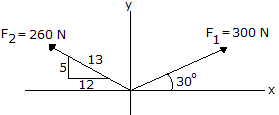Determine the magnitude and direction of the resultant force.

 A. R = 251 N,= 85.5° CCW B. R = 251 N,= 94.5° CCW C. R = 421 N,= 67.7° CCW D. R = 421 N,= 112.3° CCW

Explanation:

No answer description available for this question. Let us discuss.

17.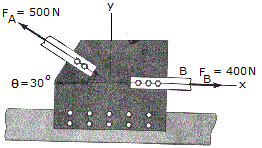The gusset plate G of a bridge joint is subjected to the two member forces at A and B. If the force at B is horizontal and the force at A is directed at= 30°, determine the magnitude and direction of the resultant force.

 A. R = 458 N,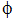= 97.5° CCW B. R = 252 N,= 82.5° CCW C. R = 252 N,= 97.5° CCW D. R = 458 N,= 82.5° CCW

Explanation:

No answer description available for this question. Let us discuss.

18.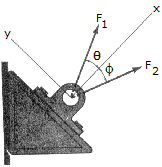If= 20° and= 35°, determine the magnitudes of F1 and F2 so that the resultant force has a magnitude of 20 lb and is directed along the positive x axis.

 A. F1 = 20.0 lb, F2 = 22.9 lb B. F1 = 25.6 lb, F2 = 26.6 lb C. F1 = 28.5 lb, F2 = 11.91 lb D. F1 = 14.00 lb, F2 = 8.35 lb

Explanation:

No answer description available for this question. Let us discuss.

19.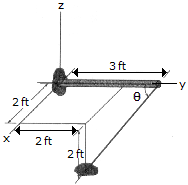Determine the anglebetween the pole AC and the wire AB.

 A.= 131.8° B.= 70.5° C.= 109.5° D.= 48.2°

Explanation:

No answer description available for this question. Let us discuss.

20.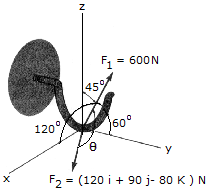Two forces act on a block. Determine the anglebetween them.

 A.= 135.0° B.= 65.1° C.= 45.0° D.= 114.9°